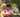### Nesting

January 31, 2020

Nesting

A string S consisting of N characters is called properly nested if:
• S is empty;
• S has the form "(U)" where U is a properly nested string;
• S has the form "VW" where V and W are properly nested strings.
For example, string "(()(())())" is properly nested but string "())" isn't.

Write a function:
function solution(S);
that, given a string S consisting of N characters, returns 1 if string S is properly nested and 0 otherwise.
For example, given S = "(()(())())", the function should return 1 and given S = "())", the function should return 0, as explained above.

Write an efficient algorithm for the following assumptions:
• N is an integer within the range [0..1,000,000];
• string S consists only of the characters "(" and/or ")".

``````function solution(S) {
// write your code in JavaScript (Node.js 8.9.4)
if(S.length<1) return 1

let L = []
let c
for(let i=0; i<S.length; i++){
if(S[i]=='(') L.push(S[i])
else { // right parenthese
if(L.length<1) return 0
c = L.pop() // matching left parenthese
}
}
if(L.length<1) return 1
else return 0 // remaining left parentheses
}``````Posted by Tai Lu ( 呂台生 ) who's building useful things.You should follow him on Twitter